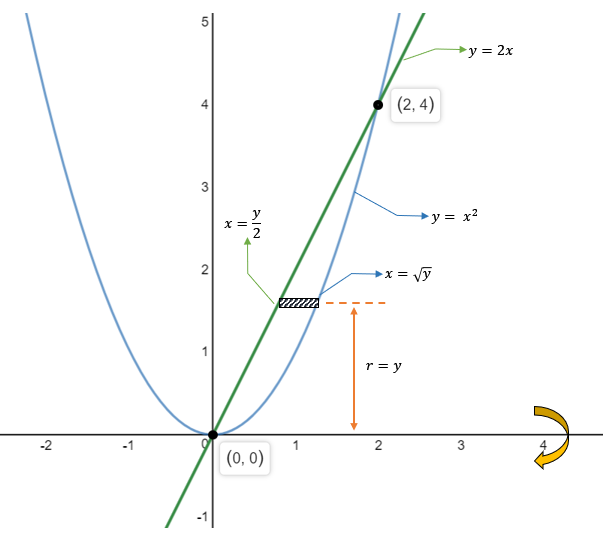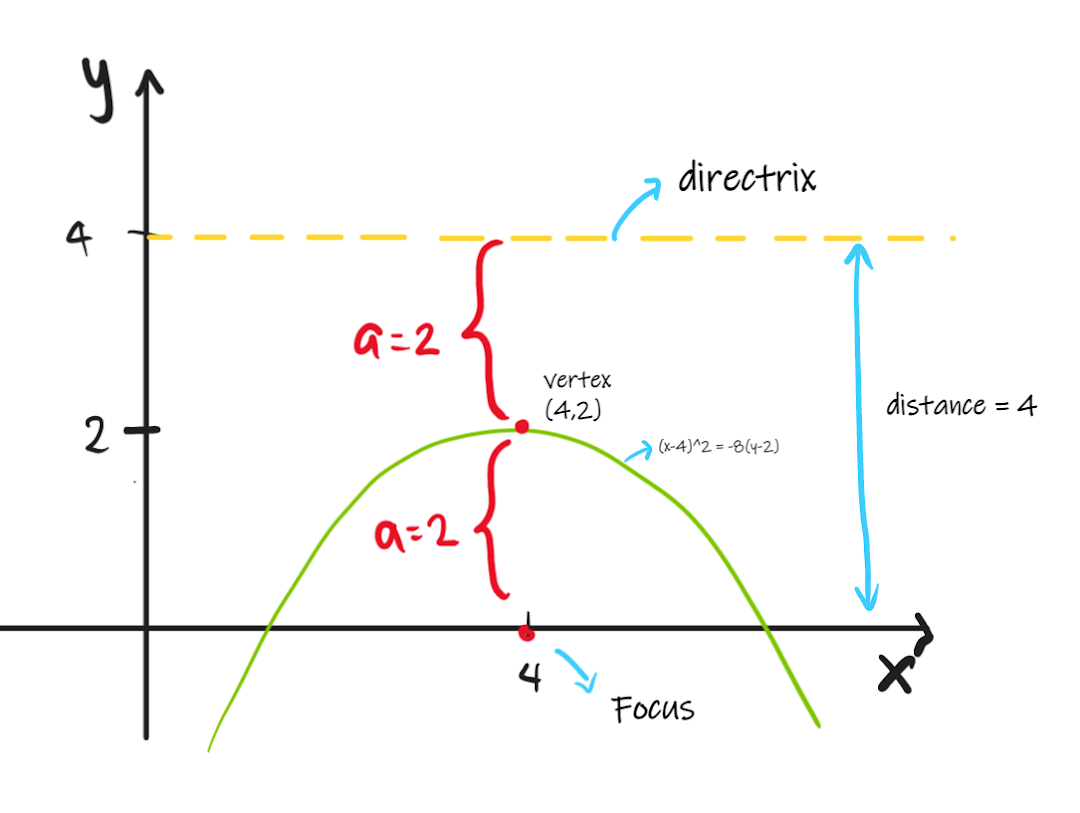## Math Series 3

1. Determine the phase shift of y = 0.5cos(0.5x-π/3).
1. π/3
2. π/2
3. 2π/5
4. 2π/3
Solution:
Equate phase angle to zero then solve for x. $$y = 0.5 \cos \left( 0.5x-\frac{\pi}{3} \right)$$ \begin{align} 0.5x-\frac{\pi}{3} & = 0 \\ x & = \frac{2 \pi}{3} \\ \end{align}

2. The function x3sinx is
1. Odd
2. Even
3. Neither
4. None
Solution:
Even functions:
$$f(x) = f(-x)$$ Odd functions: $$-f(x) = f(-x)$$
Let x = 1.1 as test value. \begin{align} f(x) & = x^3 \sin(x) \\ \\ f(1.1) & = (1.1)^3 \sin(1.1)\\ & = 1.186 \\ \\ f(-1.1) & = (-1.1)^3 \sin(1.1)\\ & = 1.186 \\ \end{align} Since $f(x) = f(-x)$, then the function is even.

3. The function cos x + sin x + tan x + cot x + sec x + csc x is
1. Both periodic and odd
2. Both periodic and even
3. Periodic but neither even nor odd
4. Not periodic
Solution:
Let x = 1.1 as test value. \begin{align} f(x) & = \cos x + \sin x + \tan x + \cot x + \sec x + \csc x \\ f(1.1) & = 7.145 \\ f(-1.1) &= -1.829 \\ \end{align} Since $f(x) \neq f(-x)$ and $-f(x) \neq f(-x)$ and all trigonometric functions are periodic, it is neither even nor odd.

4. The period of function sin 2x + cot 3x + sec 5x is
1. π
2. π/2
3. π/3
Solution:
Function Period
$y=A \sin (Bx+C)+K$
$y=A \sec (Bx+C)+K$
$T = \frac{2 \pi}{B}$
$y=A \cos (Bx+C)+K$
$y=A \csc (Bx+C)+K$
$T = \frac{2 \pi}{B}$
$y=A \tan (Bx+C)+K$
$y=A \cot (Bx+C)+K$
$T = \frac{\pi}{B}$
$$\sin 2x + \cot 3x + \sec 5x$$ $\sin 2x$: \begin{align} T_1 & = \frac{2 \pi }{2}\\ & = \pi \\ \end{align} $\cot 3x$: \begin{align} T_2 & = \frac{3 \pi }{3}\\ & = \pi \\ \end{align} $\sec 5x$: \begin{align} T_3 & = \frac{2 \pi }{5}\\ \end{align} The period of the function is the Least Common Multiple (LCM) of the periods of each term. $$LCM: T = \pi.$$
Hint: To get the LCM from the choices, divide the terms from the choices and the answer should be the least whole number.
$LCM: \frac{ \text{choices} }{ \text{given} } \equiv \text{whole number}$

5. Find the arc length of f(x) = x^2(3/2) + 2 on [1,4].
1. 7.63
2. 8.30
3. 8.01
4. 7.45
Solution:
Arc Length - Rectangular \begin{align} \mathrm{S} & = \int_{x_1}^{x_2} \sqrt{ 1 + (dy/dx)^2 } dx \\ \mathrm{S} & = \int_{y_1}^{y_2} \sqrt{ 1 + (dx/dy)^2 } dy \\ \end{align}
Solving, \begin{align} y & = x^{3/2} + 2 \text{ } [1,4] \\ \frac{dy}{dx} & = \frac{3}{2} x^{1/2} \\ \\ \mathrm{S} & = \int_1^4 \sqrt{ 1 + \left( \frac{3}{2} x^{1/2} \right)^2 } dx\\ & = 7.63 \\ \end{align}

6. Compute the arc length of the curve by the parametric equations x = 1 + cos(t) and y = sin(t) where 0 ≤ t ≤ 2π.
1. π
2. 2π/3
3. π/2
Solution:
Arc Length - Parametric \begin{align} \mathrm{S} & = \int_{t_1}^{t_2} \sqrt{ (dx/dt)^2 + (dy/dt)^2 } dt \\ \end{align}
Solving, \begin{align} x & = 1 + \cos(t) \\ dx/dt & = -\sin(t) \\ \\ y & = \sin(t)\\ dy/dt & = \cos(t)\\ \end{align} \begin{align} \mathrm{S} & = \int_0^{2 \pi} \sqrt{ (-\sin t)^2 + (\cos t)^2 } dt \\ & = 2 \pi. \\ \end{align}

7. Find the volume obtained if the region bounded by y=x^2 and y=2x is rotated about x-axis.
1. 14.5
2. 13.4
3. 11.7
4. 11.8
Solution:
Using Shell Method \begin{align} V & = 2 \pi \int_{x_1}^{x_2} rL dx \\ V & = 2 \pi \int_{y_1}^{y_2} rL dy \\ \\ V & - \text{volume} \\ r & - \text{radius or the length from the axis of rotation to the strip}\\ L & - \text{length of the strip}\\ x_1, x_2 & - \text{points of intersection at x}\\ y_1, y_2 & - \text{points of intersection at y}\\ \end{align}
Graphing,\begin{align} V & = 2 \pi \int_{y_1}^{y_2} rL dy \\ V & = 2 \pi \int_0^4 y \left( \sqrt{y} - \frac{y}{2} \right) dy \\ V & = 13.4. \\ \end{align}

8. How far is the directrix of the parabola (x-4)^2 = -8(y-2) from the x-axis?
1. x = 1
2. x = -5
3. x = 4
4. x = 0
Solution:
Standard Equation of the Parabola \begin{align} (x-h)^2 & = \pm 4a(y-k) \\ (h,k) & - \text{vertex of the parabola} \\ a & - \text{focal length or the distance from} \\ & \text{ the vertex to the focus or to the directrix} \\ \end{align}
From the equation, $(x-4)^2 = -8(y-2)$, we can take that:
• The parabola opens downward since $(-)a$.
• The focal length is a = 2.
• The vertex is (4,2).
Graphing,As can be seen from the graph, distance = 4.